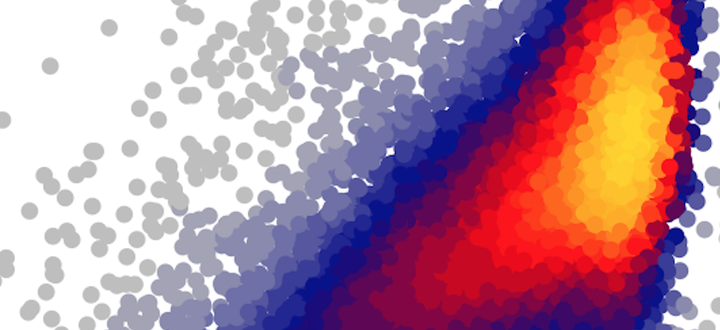# LSD Heatscatter with ggplotI love the `heatscatter()` function from the LSD R package. I’ve even used it for my own publications (see Figs. 2 and 7 in Stocker et al. 2017). Since having written this paper and creating the figures I’ve (finally) moved to using ggplot. Unfortunately, `heatscatter()` creates a plot in base R.

Googling, I found no satisfying solution. What is described on this blog does’t really do the trick.

I tried another approach. I forked the LSD library into my github and hacked an additional argument into the `heatscatter()` function as:

`ggplot` a logical: if `TRUE` uses the ggplot2 library to create plots. Defaults to `FALSE`.

When set to `TRUE`, the function returns a ggplot object. For example the following bit of code …

``````gg <- heatscatter(mdf\$lue_mod, mdf\$lue_obs, xlab = "Modelled", ylab = "Observed", ggplot = TRUE)
gg + lab(title = "Example")
``````

… prints a simple plot, by default (and hard-coded), using ggplot’s `theme_classic()`. This looks like this:

That’s it. A direct link to the hacked `heatscatter()` function is here.

Credits to the halluzinogenic LSD package developer Bjoern Schwalb!